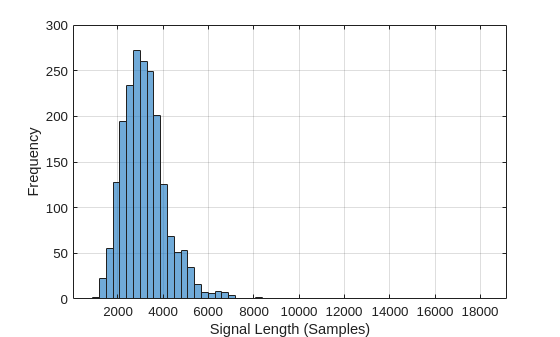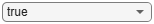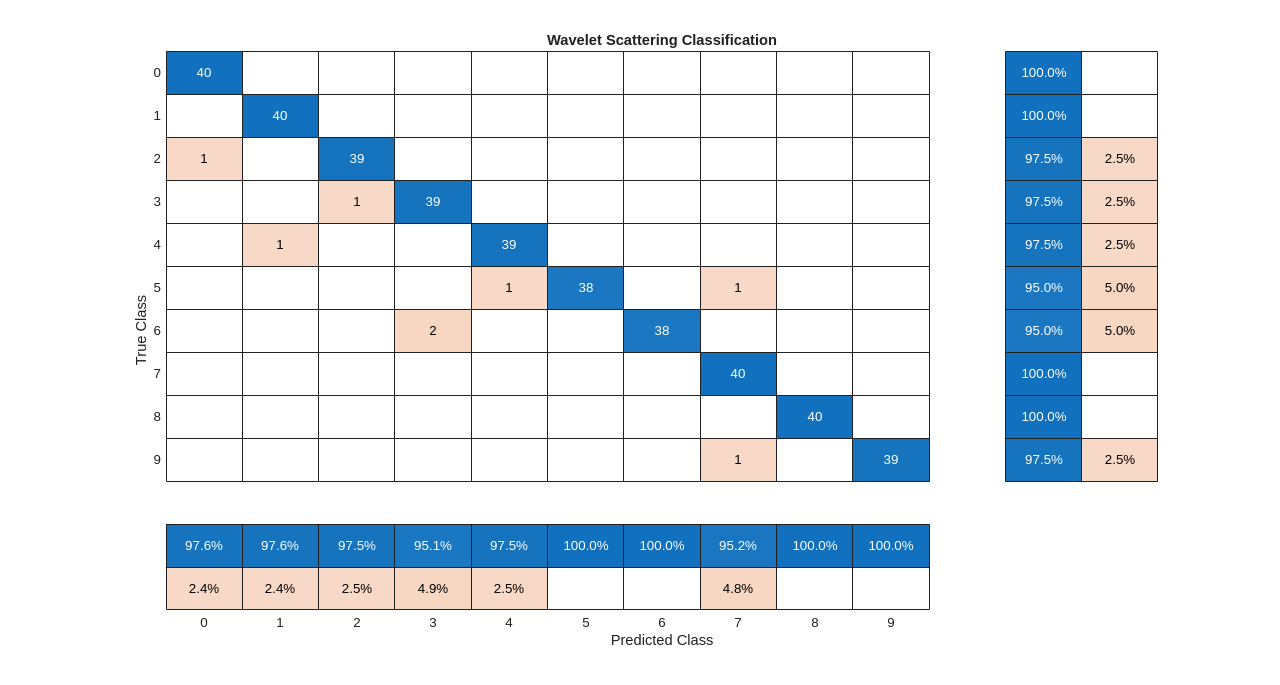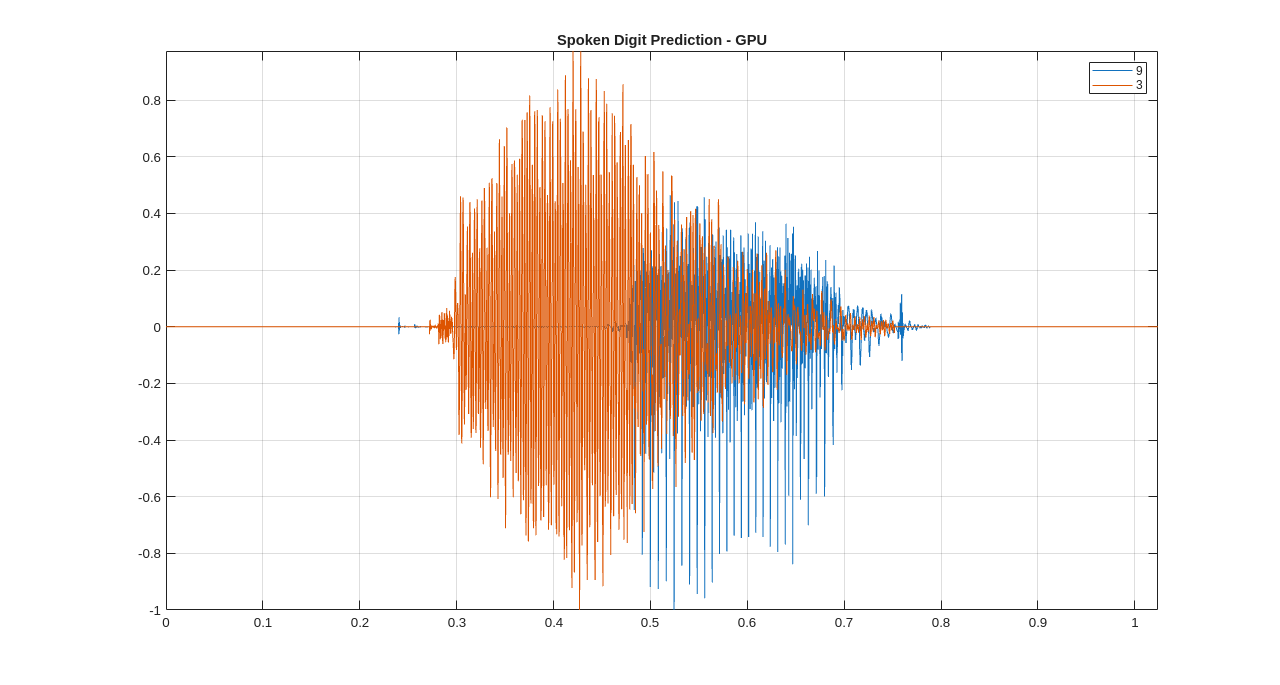# Wavelet Time Scattering with GPU Acceleration — Spoken Digit Recognition

This example shows how to accelerate the computation of wavelet scattering features using `gpuArray` (Parallel Computing Toolbox). You must have Parallel Computing Toolbox™ and a supported GPU. See GPU Computing Requirements (Parallel Computing Toolbox) for details. This example uses an NVIDIA Titan V GPU with compute capability 7.0. The section of the example that computes the scattering transform provides the option to use the GPU or CPU if you wish to compare GPU vs CPU performance.

This example reproduces the exclusively CPU version of the scattering transform found in Spoken Digit Recognition with Wavelet Scattering and Deep Learning.

### Data

Clone or download the Free Spoken Digit Dataset (FSDD), available at `https://github.com/Jakobovski/free-spoken-digit-dataset`. FSDD is an open data set, which means that it can grow over time. This example uses the version committed on 08/20/2020 which consists of 3000 recordings of the English digits 0 through 9 obtained from six speakers. The data is sampled at 8000 Hz.

Use `audioDatastore` to manage data access and ensure random division of the recordings into training and test sets. Set the `location` property to the location of the FSDD recordings folder on your computer. In this example, the data is stored in a folder under `tempdir`.

```location = fullfile(tempdir,'free-spoken-digit-dataset','recordings'); ads = audioDatastore(location);```

The helper function, `helpergenLabels`, defined at the end of this example, creates a categorical array of labels from the FSDD files. List the classes and the number of examples in each class.

```ads.Labels = helpergenLabels(ads); summary(ads.Labels)```
``` 0 300 1 300 2 300 3 300 4 300 5 300 6 300 7 300 8 300 9 300 ```

The FSDD dataset consists of 10 balanced classes with 300 recordings each. The recordings in the FSDD are not of equal duration. Read through the FSDD files and construct a histogram of the signal lengths.

```LenSig = zeros(numel(ads.Files),1); nr = 1; while hasdata(ads) digit = read(ads); LenSig(nr) = numel(digit); nr = nr+1; end reset(ads) histogram(LenSig) grid on xlabel('Signal Length (Samples)') ylabel('Frequency')```The histogram shows that the distribution of recording lengths is positively skewed. For classification, this example uses a common signal length of 8192 samples. The value 8192, a conservative choice, ensures that truncating longer recordings does not affect (cut off) the speech content. If the signal is greater than 8192 samples, or 1.024 seconds, in length, the recording is truncated to 8192 samples. If the signal is less than 8192 samples in length, the signal is symmetrically prepended and appended with zeros out to a length of 8192 samples.

### Wavelet Time Scattering

Create a wavelet time scattering network using an invariant scale of 0.22 seconds. Because the feature vectors will be created by averaging the scattering transform over all time samples, set the `OversamplingFactor` to 2. Setting the value to 2 will result in a four-fold increase in the number of scattering coefficients for each path with respect to the critically downsampled value.

```sn = waveletScattering('SignalLength',8192,'InvarianceScale',0.22,... 'SamplingFrequency',8000,'OversamplingFactor',2);```

The settings of the scattering network results in 326 paths. You can verify this with the following code.

```[~,npaths] = paths(sn); sum(npaths)```
```ans = 326 ```

Split the FSDD into training and test sets. Allocate 80% of the data to the training set and retain 20% for the test set. The training data is for training the classifier based on the scattering transform. The test data is for assessing the model's ability to generalize to unseen data.

```rng default; ads = shuffle(ads); [adsTrain,adsTest] = splitEachLabel(ads,0.8); summary(adsTrain.Labels)```
``` 0 240 1 240 2 240 3 240 4 240 5 240 6 240 7 240 8 240 9 240 ```
`summary(adsTest.Labels)`
``` 0 60 1 60 2 60 3 60 4 60 5 60 6 60 7 60 8 60 9 60 ```

Form a 8192-by-2400 matrix where each column is a spoken-digit recording. The helper function `helperReadSPData` truncates or pads the data to length 8192 and normalizes each record by its maximum value. The helper function casts the data to single precision.

```Xtrain = []; scatds_Train = transform(adsTrain,@(x)helperReadSPData(x)); while hasdata(scatds_Train) smat = read(scatds_Train); Xtrain = cat(2,Xtrain,smat); end```

Repeat the process for the held-out test set. The resulting matrix is 8192-by-600.

```Xtest = []; scatds_Test = transform(adsTest,@(x)helperReadSPData(x)); while hasdata(scatds_Test) smat = read(scatds_Test); Xtest = cat(2,Xtest,smat); end```

Apply the scattering transform to the training and test sets. Move both the training and test data sets to the GPU using `gpuArray`. The use of `gpuArray` with a CUDA-enabled NVIDIA GPU provides a significant acceleration. With this scattering network, batch size, and GPU, the GPU implementation computes the scattering features approximately 15 times faster than the CPU version. If you do not wish to use the GPU, set `useGPU` to `false`. You can also alternate the value of `useGPU `to compare GPU vs CPU performance.

```useGPU =true; if useGPU Xtrain = gpuArray(Xtrain); Strain = sn.featureMatrix(Xtrain); Xtrain = gather(Xtrain); Xtest = gpuArray(Xtest); Stest = sn.featureMatrix(Xtest); Xtest = gather(Xtest); else Strain = sn.featureMatrix(Xtrain); Stest = sn.featureMatrix(Xtest); end```

Obtain the scattering features for the training and test sets.

```TrainFeatures = Strain(2:end,:,:); TrainFeatures = squeeze(mean(TrainFeatures,2))'; TestFeatures = Stest(2:end,:,:); TestFeatures = squeeze(mean(TestFeatures,2))';```

This example uses a support vector machine (SVM) classifier with a quadratic polynomial kernel. Fit the SVM model to the scattering features.

```template = templateSVM(... 'KernelFunction', 'polynomial', ... 'PolynomialOrder', 2, ... 'KernelScale', 'auto', ... 'BoxConstraint', 1, ... 'Standardize', true); classificationSVM = fitcecoc(... TrainFeatures, ... adsTrain.Labels, ... 'Learners', template, ... 'Coding', 'onevsone', ... 'ClassNames', categorical({'0'; '1'; '2'; '3'; '4'; '5'; '6'; '7'; '8'; '9'}));```

Use k-fold cross-validation to predict the generalization accuracy of the model. Split the training set into five groups for cross-validation.

```partitionedModel = crossval(classificationSVM, 'KFold', 5); [validationPredictions, validationScores] = kfoldPredict(partitionedModel); validationAccuracy = (1 - kfoldLoss(partitionedModel, 'LossFun', 'ClassifError'))*100```
```validationAccuracy = 97.2500 ```

The estimated generalization accuracy is approximately 97%. Now use the SVM model to predict the held-out test set.

```predLabels = predict(classificationSVM,TestFeatures); testAccuracy = sum(predLabels==adsTest.Labels)/numel(predLabels)*100```
```testAccuracy = 97 ```

The accuracy is also approximately 97% on the held-out test set.

Summarize the performance of the model on the test set with a confusion chart. Display the precision and recall for each class by using column and row summaries. The table at the bottom of the confusion chart shows the precision values for each class. The table to the right of the confusion chart shows the recall values.

```figure('Units','normalized','Position',[0.2 0.2 0.5 0.5]); ccscat = confusionchart(adsTest.Labels,predLabels); ccscat.Title = 'Wavelet Scattering Classification'; ccscat.ColumnSummary = 'column-normalized'; ccscat.RowSummary = 'row-normalized';```As a final example, read the first two records from the dataset, calculate the scattering features, and predict the spoken digit using the SVM trained with scattering features.

```reset(ads); sig1 = helperReadSPData(read(ads)); scat1 = sn.featureMatrix(sig1); scat1 = mean(scat1(2:end,:),2)'; plab1 = predict(classificationSVM,scat1);```

Read the next record and predict the digit.

```sig2 = helperReadSPData(read(ads)); scat2 = sn.featureMatrix(sig2); scat2 = mean(scat2(2:end,:),2)'; plab2 = predict(classificationSVM,scat2);```
```t = 0:1/8000:(8192*1/8000)-1/8000; plot(t,[sig1 sig2]) grid on axis tight legend(char(plab1),char(plab2)) title('Spoken Digit Prediction - GPU')```### Appendix

The following helper functions are used in this example.

helpergenLabels — generates labels based on the file names in the FSDD.

```function Labels = helpergenLabels(ads) % This function is only for use in Wavelet Toolbox examples. It may be % changed or removed in a future release. tmp = cell(numel(ads.Files),1); expression = "[0-9]+_"; for nf = 1:numel(ads.Files) idx = regexp(ads.Files{nf},expression); tmp{nf} = ads.Files{nf}(idx); end Labels = categorical(tmp); end```

helperReadSPData — Ensures that each spoken-digit recording is 8192 samples long.

```function x = helperReadSPData(x) % This function is only for use Wavelet Toolbox examples. It may change or % be removed in a future release. N = numel(x); if N > 8192 x = x(1:8192); elseif N < 8192 pad = 8192-N; prepad = floor(pad/2); postpad = ceil(pad/2); x = [zeros(prepad,1) ; x ; zeros(postpad,1)]; end x = single(x./max(abs(x))); end```